# Trajectory Pattern Mining NTU IM Hsieh HsunPing Trajectory

• Slides: 37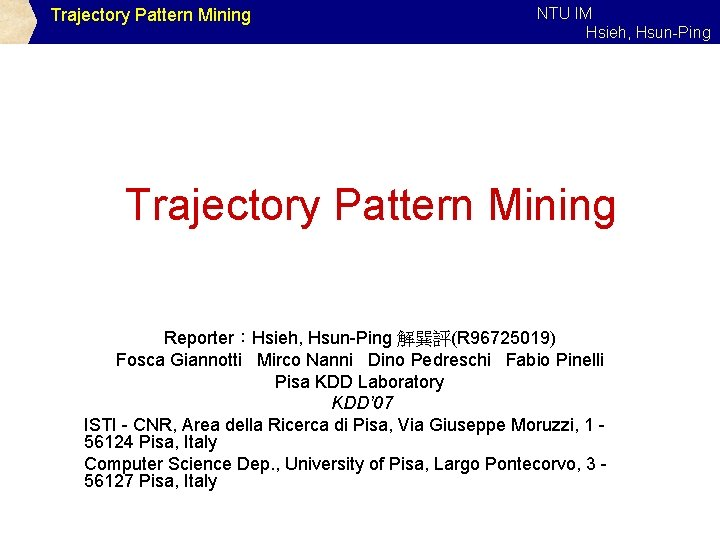Trajectory Pattern Mining NTU IM Hsieh, Hsun-Ping Trajectory Pattern Mining Reporter：Hsieh, Hsun-Ping 解巽評(R 96725019) Fosca Giannotti Mirco Nanni Dino Pedreschi Fabio Pinelli Pisa KDD Laboratory KDD’ 07 ISTI - CNR, Area della Ricerca di Pisa, Via Giuseppe Moruzzi, 1 56124 Pisa, Italy Computer Science Dep. , University of Pisa, Largo Pontecorvo, 3 56127 Pisa, Italy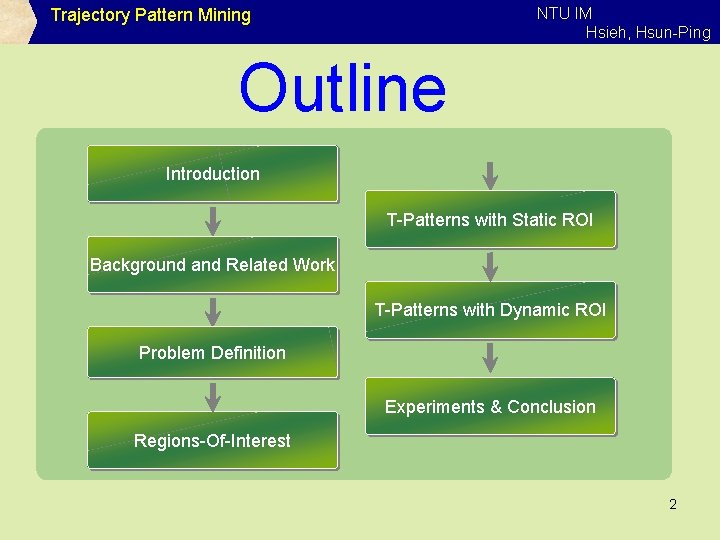NTU IM Hsieh, Hsun-Ping Trajectory Pattern Mining Outline Introduction T-Patterns with Static ROI Background and Related Work T-Patterns with Dynamic ROI Problem Definition Experiments & Conclusion Regions-Of-Interest 2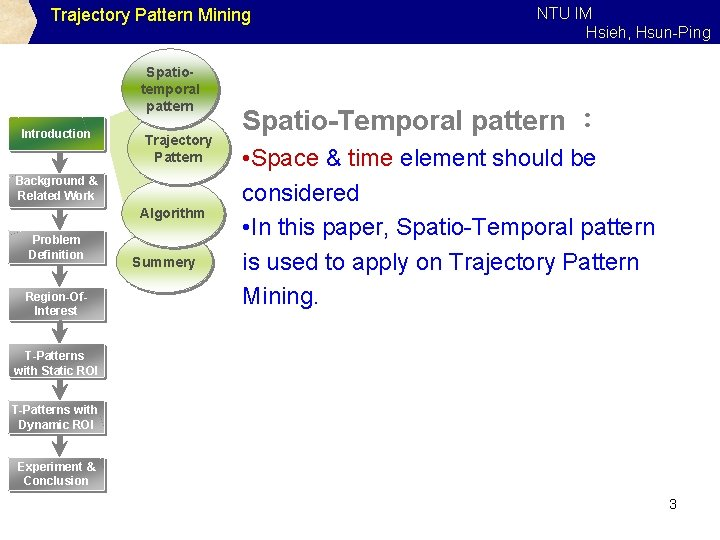Trajectory Pattern Mining Spatiotemporal pattern Introduction Trajectory Pattern Background & Related Work Algorithm Problem Definition Region-Of. Interest Summery NTU IM Hsieh, Hsun-Ping Spatio-Temporal pattern ： • Space & time element should be considered • In this paper, Spatio-Temporal pattern is used to apply on Trajectory Pattern Mining. T-Patterns with Static ROI T-Patterns with Dynamic ROI Experiment & Conclusion 3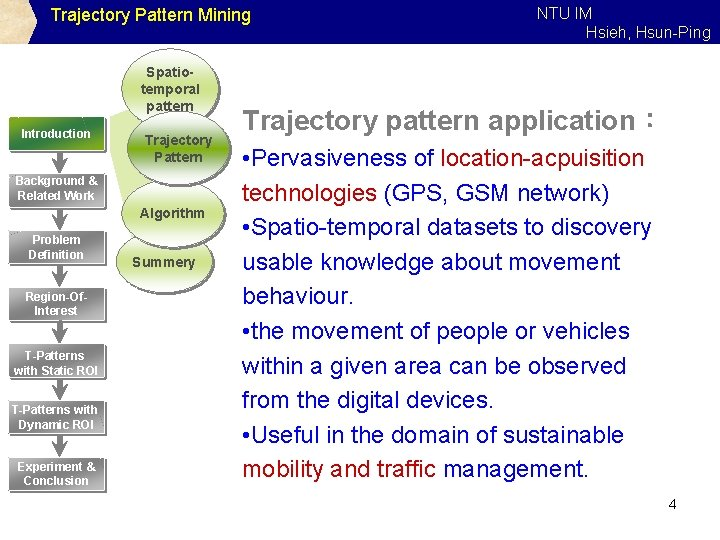Trajectory Pattern Mining Spatiotemporal pattern Introduction Trajectory Pattern Background & Related Work Algorithm Problem Definition Region-Of. Interest T-Patterns with Static ROI T-Patterns with Dynamic ROI Experiment & Conclusion Summery NTU IM Hsieh, Hsun-Ping Trajectory pattern application： • Pervasiveness of location-acpuisition technologies (GPS, GSM network) • Spatio-temporal datasets to discovery usable knowledge about movement behaviour. • the movement of people or vehicles within a given area can be observed from the digital devices. • Useful in the domain of sustainable mobility and traffic management. 4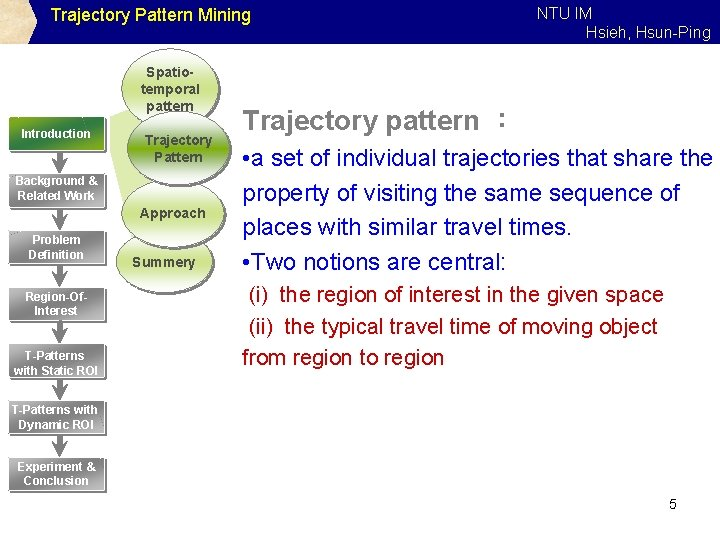Trajectory Pattern Mining Spatiotemporal pattern Introduction Trajectory Pattern Background & Related Work Approach Problem Definition Region-Of. Interest T-Patterns with Static ROI Summery NTU IM Hsieh, Hsun-Ping Trajectory pattern ： • a set of individual trajectories that share the property of visiting the same sequence of places with similar travel times. • Two notions are central: (i) the region of interest in the given space (ii) the typical travel time of moving object from region to region T-Patterns with Dynamic ROI Experiment & Conclusion 5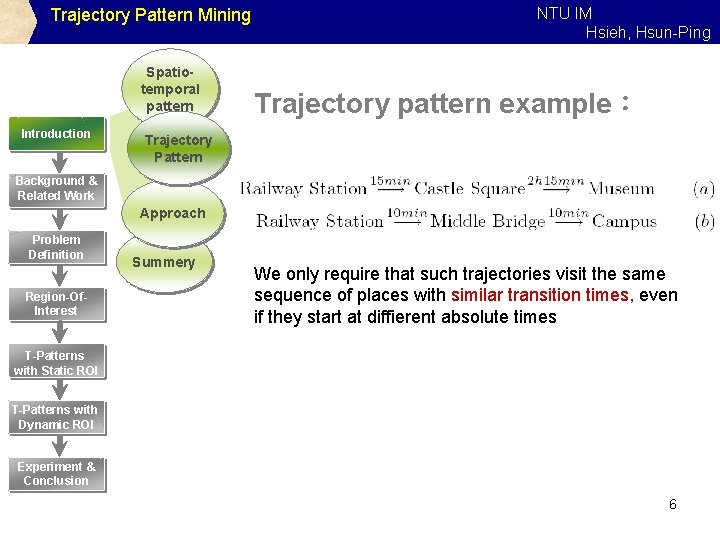Trajectory Pattern Mining Spatiotemporal pattern Introduction NTU IM Hsieh, Hsun-Ping Trajectory pattern example： Trajectory Pattern Background & Related Work Approach Problem Definition Region-Of. Interest Summery We only require that such trajectories visit the same sequence of places with similar transition times, even if they start at diffierent absolute times T-Patterns with Static ROI T-Patterns with Dynamic ROI Experiment & Conclusion 6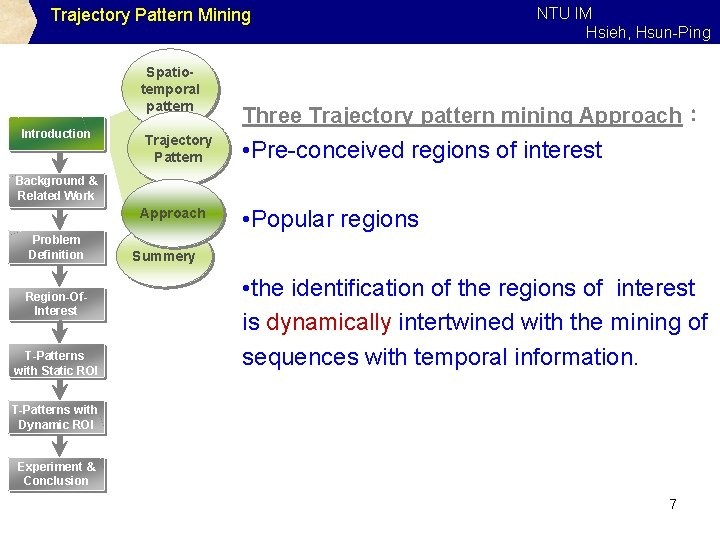Trajectory Pattern Mining Spatiotemporal pattern Introduction Trajectory Pattern NTU IM Hsieh, Hsun-Ping Three Trajectory pattern mining Approach： • Pre-conceived regions of interest Background & Related Work Approach Problem Definition Region-Of. Interest T-Patterns with Static ROI • Popular regions Summery • the identification of the regions of interest is dynamically intertwined with the mining of sequences with temporal information. T-Patterns with Dynamic ROI Experiment & Conclusion 7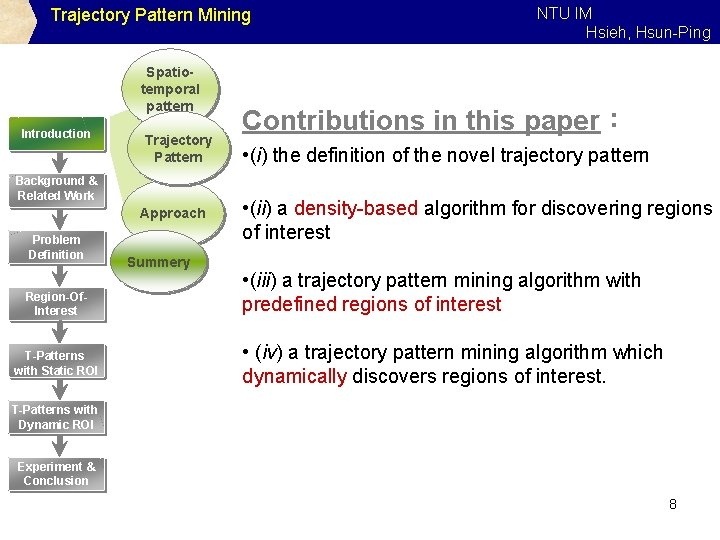Trajectory Pattern Mining Spatiotemporal pattern Introduction Trajectory Pattern Background & Related Work Approach Problem Definition Region-Of. Interest T-Patterns with Static ROI Summery NTU IM Hsieh, Hsun-Ping Contributions in this paper： • (i) the definition of the novel trajectory pattern • (ii) a density-based algorithm for discovering regions of interest • (iii) a trajectory pattern mining algorithm with predefined regions of interest • (iv) a trajectory pattern mining algorithm which dynamically discovers regions of interest. T-Patterns with Dynamic ROI Experiment & Conclusion 8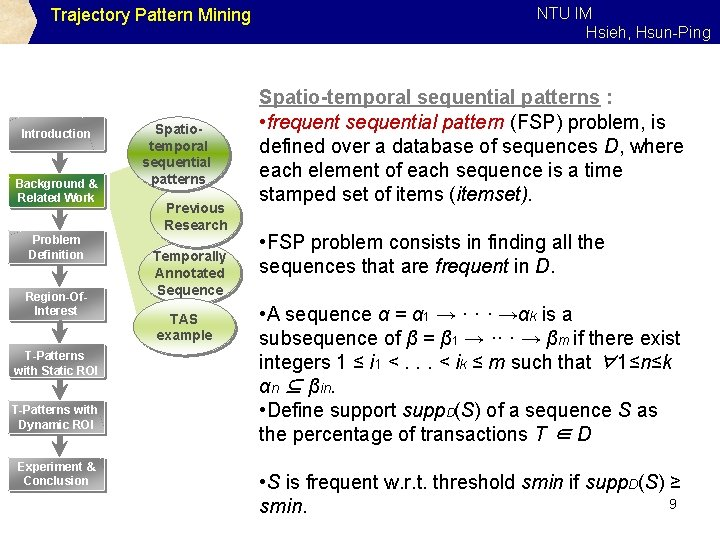Trajectory Pattern Mining Introduction Background & Related Work Problem Definition Region-Of. Interest T-Patterns with Static ROI T-Patterns with Dynamic ROI Experiment & Conclusion Spatiotemporal sequential patterns Previous Research Temporally Annotated Sequence TAS example NTU IM Hsieh, Hsun-Ping Spatio-temporal sequential patterns : • frequent sequential pattern (FSP) problem, is defined over a database of sequences D, where each element of each sequence is a time stamped set of items (itemset). • FSP problem consists in finding all the sequences that are frequent in D. • A sequence α = α 1 → · · · →αk is a subsequence of β = β 1 → ·· · → βm if there exist integers 1 ≤ i 1 <. . . < ik ≤ m such that ∀1≤n≤k αn ⊆ βin. • Define support supp. D(S) of a sequence S as the percentage of transactions T ∈ D • S is frequent w. r. t. threshold smin if supp. D(S) ≥ 9 smin.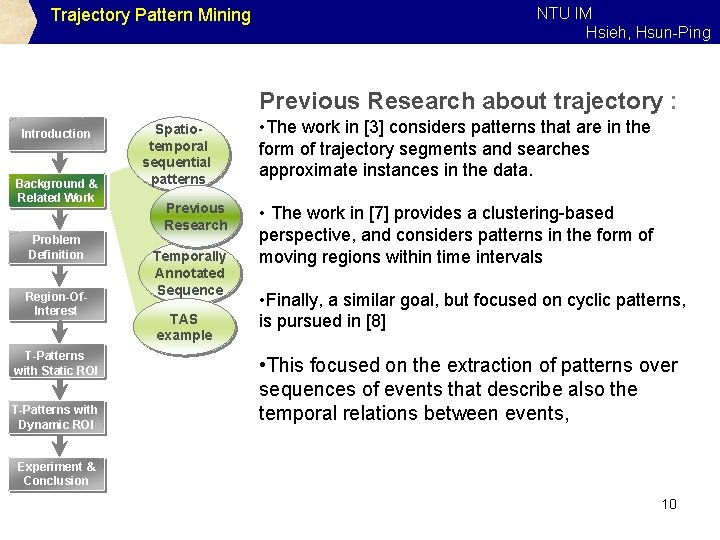Trajectory Pattern Mining NTU IM Hsieh, Hsun-Ping Previous Research about trajectory : Introduction Background & Related Work Problem Definition Region-Of. Interest T-Patterns with Static ROI T-Patterns with Dynamic ROI Spatiotemporal sequential patterns Previous Research Temporally Annotated Sequence TAS example • The work in  considers patterns that are in the form of trajectory segments and searches approximate instances in the data. • The work in  provides a clustering-based perspective, and considers patterns in the form of moving regions within time intervals • Finally, a similar goal, but focused on cyclic patterns, is pursued in  • This focused on the extraction of patterns over sequences of events that describe also the temporal relations between events, Experiment & Conclusion 10NTU IM Hsieh, Hsun-Ping Trajectory Pattern Mining Temporally Annotated Sequence : Introduction Background & Related Work Problem Definition Region-Of. Interest Spatiotemporal sequential patterns • Temporally annotated sequences (TAS), introduced in , are an extension of sequential patterns that enrich sequences with information about the typical transition times between their elements. Previous Research Temporally Annotated Sequence TAS example • Example: T-Patterns with Static ROI T-Patterns with Dynamic ROI Experiment & Conclusion 11NTU IM Hsieh, Hsun-Ping Trajectory Pattern Mining Definition 1 : Introduction Background & Related Work Problem Definition Region-Of. Interest Spatiotemporal sequential patterns Previous Research Temporally Annotated Sequence TAS example T-Patterns with Static ROI T-Patterns with Dynamic ROI Experiment & Conclusion 12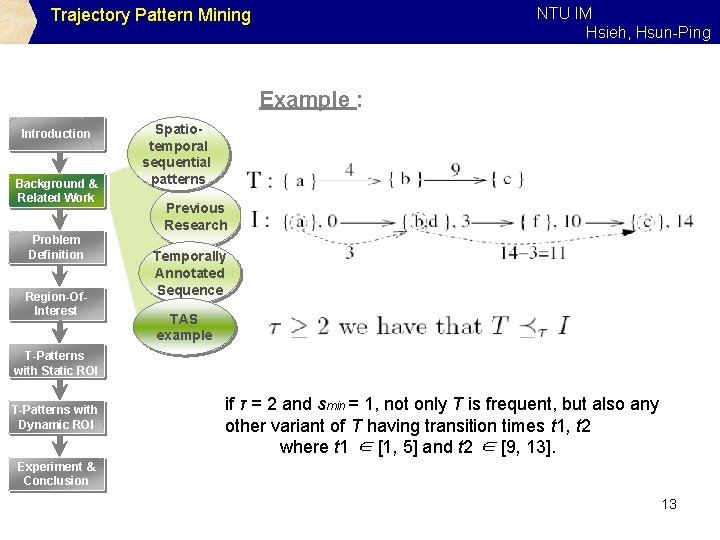NTU IM Hsieh, Hsun-Ping Trajectory Pattern Mining Example : Introduction Background & Related Work Problem Definition Region-Of. Interest Spatiotemporal sequential patterns Previous Research Temporally Annotated Sequence TAS example T-Patterns with Static ROI T-Patterns with Dynamic ROI if τ = 2 and smin = 1, not only T is frequent, but also any other variant of T having transition times t 1, t 2 where t 1 ∈ [1, 5] and t 2 ∈ [9, 13]. Experiment & Conclusion 13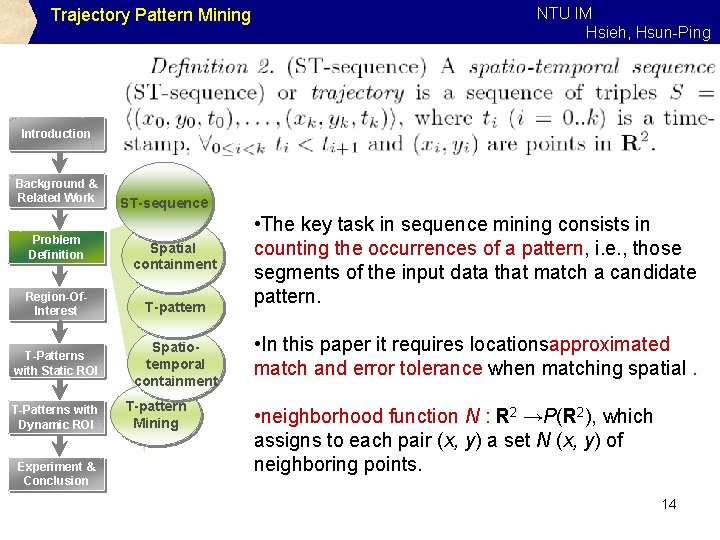Trajectory Pattern Mining NTU IM Hsieh, Hsun-Ping Introduction Background & Related Work Problem Definition ST-sequence Spatial containment Region-Of. Interest T-pattern T-Patterns with Static ROI Spatiotemporal containment T-Patterns with Dynamic ROI Experiment & Conclusion T-pattern Mining • The key task in sequence mining consists in counting the occurrences of a pattern, i. e. , those segments of the input data that match a candidate pattern. • In this paper it requires locationsapproximated match and error tolerance when matching spatial. • neighborhood function N : R 2 →P(R 2), which assigns to each pair (x, y) a set N (x, y) of neighboring points. 14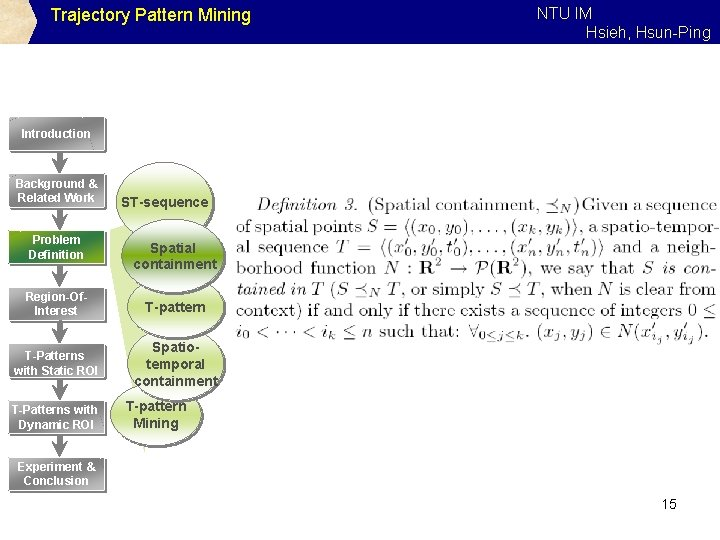Trajectory Pattern Mining NTU IM Hsieh, Hsun-Ping Introduction Background & Related Work Problem Definition ST-sequence Spatial containment Region-Of. Interest T-pattern T-Patterns with Static ROI Spatiotemporal containment T-Patterns with Dynamic ROI T-pattern Mining Experiment & Conclusion 15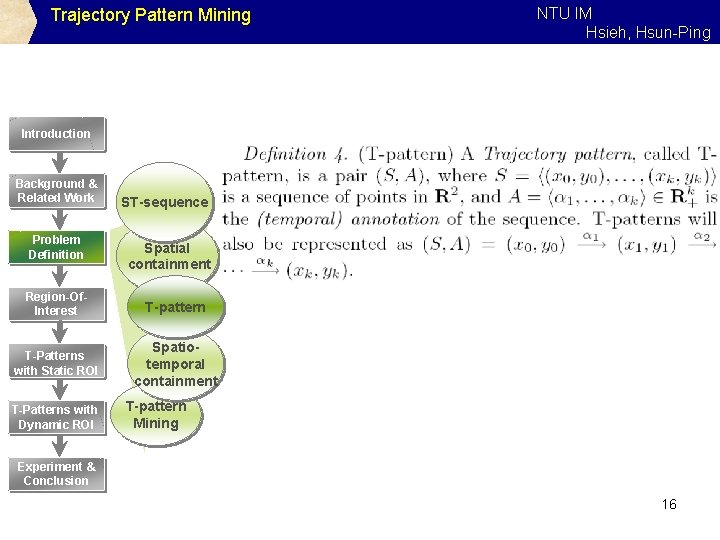Trajectory Pattern Mining NTU IM Hsieh, Hsun-Ping Introduction Background & Related Work Problem Definition ST-sequence Spatial containment Region-Of. Interest T-pattern T-Patterns with Static ROI Spatiotemporal containment T-Patterns with Dynamic ROI T-pattern Mining Experiment & Conclusion 16Trajectory Pattern Mining NTU IM Hsieh, Hsun-Ping Introduction Background & Related Work Problem Definition ST-sequence Spatial containment Region-Of. Interest T-pattern T-Patterns with Static ROI Spatiotemporal containment T-Patterns with Dynamic ROI T-pattern Mining Experiment & Conclusion 17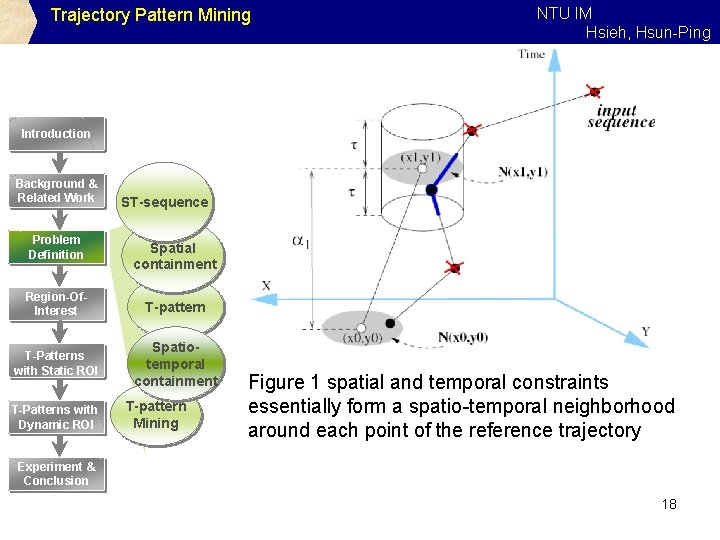Trajectory Pattern Mining NTU IM Hsieh, Hsun-Ping Introduction Background & Related Work Problem Definition ST-sequence Spatial containment Region-Of. Interest T-pattern T-Patterns with Static ROI Spatiotemporal containment T-Patterns with Dynamic ROI T-pattern Mining Figure 1 spatial and temporal constraints essentially form a spatio-temporal neighborhood around each point of the reference trajectory Experiment & Conclusion 18Trajectory Pattern Mining NTU IM Hsieh, Hsun-Ping Introduction Background & Related Work Problem Definition ST-sequence Spatial containment Region-Of. Interest T-pattern T-Patterns with Static ROI Spatiotemporal containment T-Patterns with Dynamic ROI T-pattern Mining Experiment & Conclusion 19NTU IM Hsieh, Hsun-Ping Trajectory Pattern Mining REGIONS-OF-INTEREST neighborhood function is used to model Regions-of. Interest (Ro. I), that represent a natural way to partition the space into meaningful areas and to associate spatial points with region labels. Introduction Background & Related Work Problem Definition Region-Of. Interest T-Patterns with Static ROI T-Patterns with Dynamic ROI Experiment & Conclusion Integrating ROI & trajectories : Region-Of. Interest • Input: set R of disjoint spatial regions • Neigborhood function: Trajectory Preproceing Discovering ROI Popualr points detection ROI Construction • two points are considered similar iff they fall in the same region. • points disregarded by R will be virtually deleted from trajectories and spatio-temporal patterns. 20Trajectory Pattern Mining NTU IM Hsieh, Hsun-Ping Introduction Background & Related Work Problem Definition Region-Of. Interest Trajectory Preprocessing T-Patterns with Static ROI Discovering ROI T-Patterns with Dynamic ROI Popular points detection Experiment & Conclusion • The regions associated with each point, i. e. , NR(x, y), are essentially used as labels representing events of the form “the trajectory is in region NR(x, y) at time t” • the methods developed for extracting frequent TAS’s can be directly applied to the translated input sequences, and each pattern (TAS) of the form A →B represents the set of T-patterns ROI Construction 21Trajectory Pattern Mining NTU IM Hsieh, Hsun-Ping Static preprocessed spatial regions Introduction When Regions-of-Interest are not provided by external means they have to be automatically computed through some heuristics. Background & Related Work Problem Definition Region-Of. Interest Trajectory Preproceing T-Patterns with Static ROI Discovering ROI T-Patterns with Dynamic ROI Popualr points detection Experiment & Conclusion The underlying idea is that locations frequently visited by moving objects probably represent interesting places consideration the density of spatial regions. ROI Construction 22Trajectory Pattern Mining NTU IM Hsieh, Hsun-Ping Trajectory preprocessing • Assuming to know a suitable set of Ro. I, applying them to the T-pattern mining problem simply consists in preprocessing the input sequences to corresponding sequences of Ro. I. Introduction Background & Related Work Problem Definition Region-Of. Interest Trajectory Preprocessing T-Patterns with Static ROI Discovering ROI T-Patterns with Dynamic ROI Popular points detection Experiment & Conclusion ROI Construction • provide a model for such point movement, e. g. , linear regression. it is not obvious which time-stamp should be associated with the event “Region A” in the translated sequence. • Different way in this paper: 1. if the trajectory starts at time t from a point already inside a region A, yield the couple (A, t); 2. An object can enter several times in a region, and each entry will be associated with a different 23 time-stamp.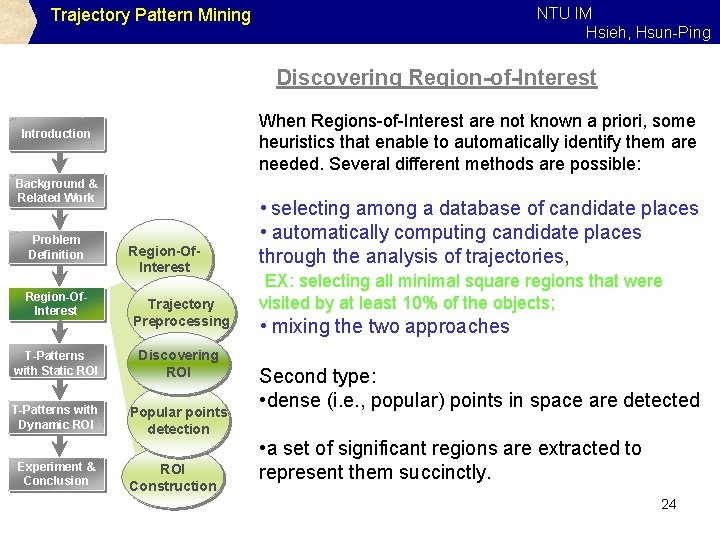NTU IM Hsieh, Hsun-Ping Trajectory Pattern Mining Discovering Region-of-Interest When Regions-of-Interest are not known a priori, some heuristics that enable to automatically identify them are needed. Several different methods are possible: Introduction Background & Related Work Problem Definition Region-Of. Interest Trajectory Preprocessing T-Patterns with Static ROI Discovering ROI T-Patterns with Dynamic ROI Popular points detection Experiment & Conclusion ROI Construction • selecting among a database of candidate places • automatically computing candidate places through the analysis of trajectories, EX: selecting all minimal square regions that were visited by at least 10% of the objects; • mixing the two approaches Second type: • dense (i. e. , popular) points in space are detected • a set of significant regions are extracted to represent them succinctly. 24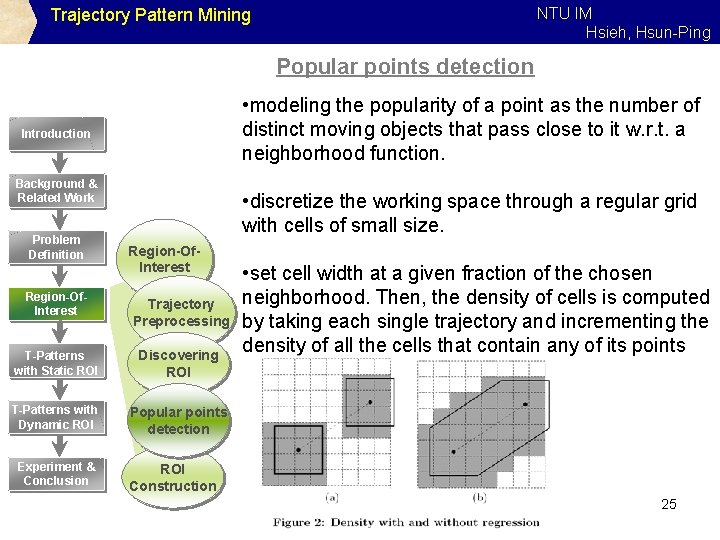NTU IM Hsieh, Hsun-Ping Trajectory Pattern Mining Popular points detection • modeling the popularity of a point as the number of distinct moving objects that pass close to it w. r. t. a neighborhood function. Introduction Background & Related Work Problem Definition Region-Of. Interest • discretize the working space through a regular grid with cells of small size. Region-Of. Interest Trajectory Preprocessing T-Patterns with Static ROI Discovering ROI T-Patterns with Dynamic ROI Popular points detection Experiment & Conclusion • set cell width at a given fraction of the chosen neighborhood. Then, the density of cells is computed by taking each single trajectory and incrementing the density of all the cells that contain any of its points ROI Construction 25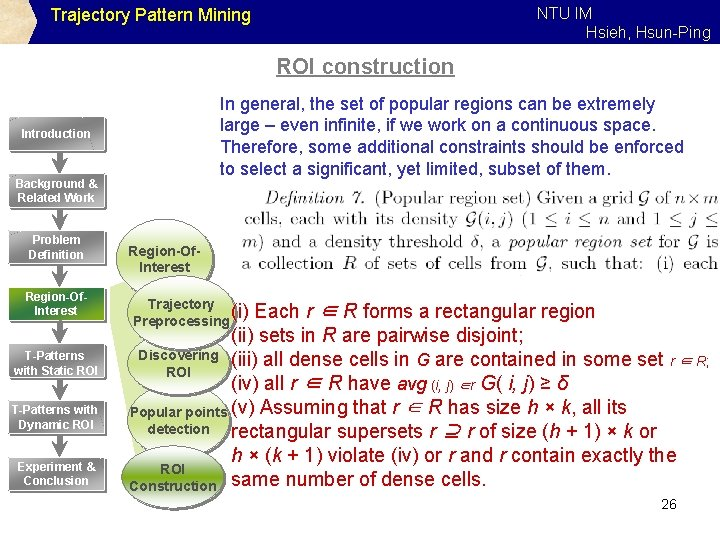NTU IM Hsieh, Hsun-Ping Trajectory Pattern Mining ROI construction In general, the set of popular regions can be extremely large – even infinite, if we work on a continuous space. Therefore, some additional constraints should be enforced to select a significant, yet limited, subset of them. Introduction Background & Related Work Problem Definition Region-Of. Interest T-Patterns with Static ROI T-Patterns with Dynamic ROI Experiment & Conclusion Region-Of. Interest Trajectory (i) Preprocessing Each r ∈ R forms a rectangular region (ii) sets in R are pairwise disjoint; Discovering (iii) all dense cells in G are contained in some set r ∈ R; ROI (iv) all r ∈ R have avg (i, j) ∈r G( i, j) ≥ δ Popular points (v) Assuming that r ∈ R has size h × k, all its detection rectangular supersets r ⊇ r of size (h + 1) × k or h × (k + 1) violate (iv) or r and r contain exactly the ROI Construction same number of dense cells. 26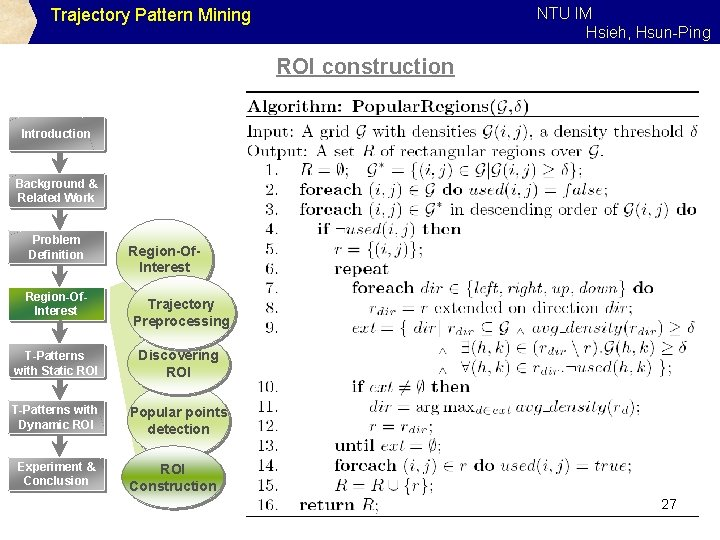NTU IM Hsieh, Hsun-Ping Trajectory Pattern Mining ROI construction Introduction Background & Related Work Problem Definition Region-Of. Interest Trajectory Preprocessing T-Patterns with Static ROI Discovering ROI T-Patterns with Dynamic ROI Popular points detection Experiment & Conclusion ROI Construction 27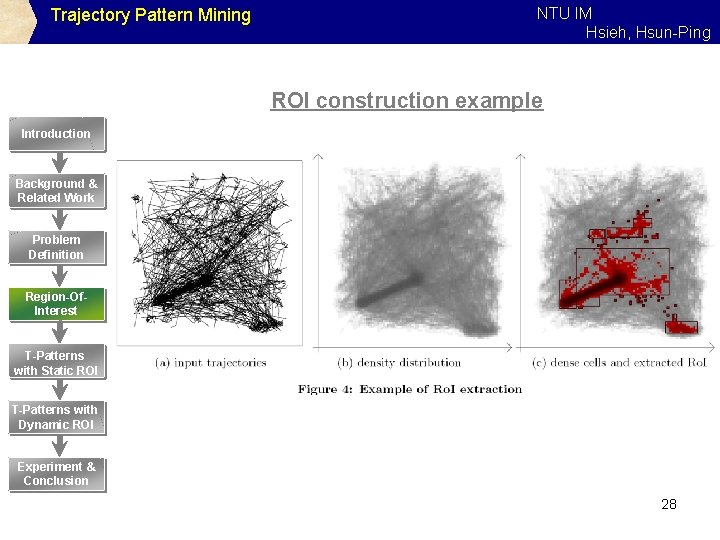Trajectory Pattern Mining NTU IM Hsieh, Hsun-Ping ROI construction example Introduction Background & Related Work Problem Definition Region-Of. Interest T-Patterns with Static ROI T-Patterns with Dynamic ROI Experiment & Conclusion 28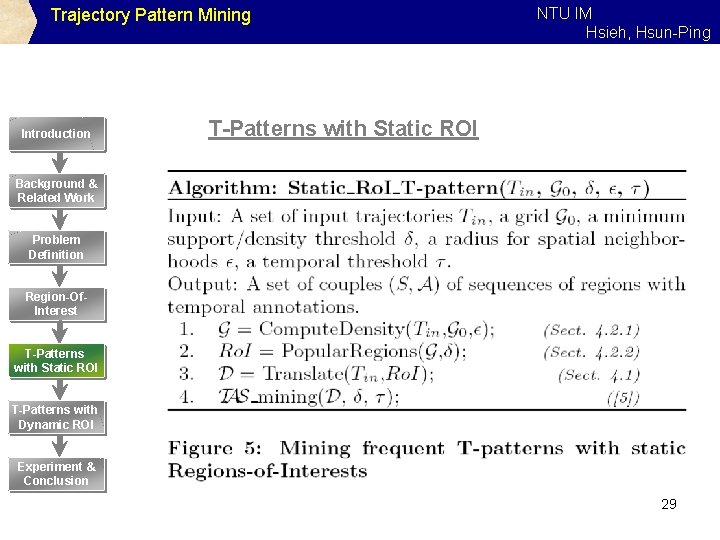Trajectory Pattern Mining Introduction NTU IM Hsieh, Hsun-Ping T-Patterns with Static ROI Background & Related Work Problem Definition Region-Of. Interest T-Patterns with Static ROI T-Patterns with Dynamic ROI Experiment & Conclusion 29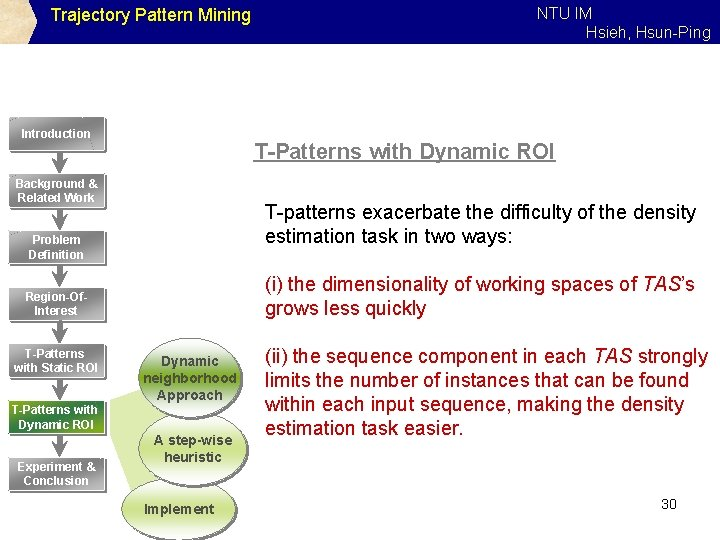Trajectory Pattern Mining Introduction T-Patterns with Dynamic ROI Background & Related Work T-patterns exacerbate the difficulty of the density estimation task in two ways: Problem Definition (i) the dimensionality of working spaces of TAS’s grows less quickly Region-Of. Interest T-Patterns with Static ROI Dynamic neighborhood Approach T-Patterns with Dynamic ROI Experiment & Conclusion NTU IM Hsieh, Hsun-Ping A step-wise heuristic Implement (ii) the sequence component in each TAS strongly limits the number of instances that can be found within each input sequence, making the density estimation task easier. 30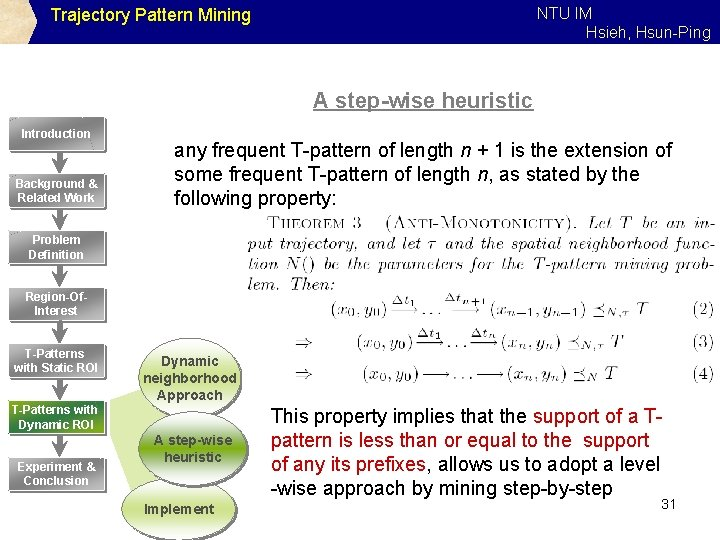NTU IM Hsieh, Hsun-Ping Trajectory Pattern Mining A step-wise heuristic Introduction Background & Related Work any frequent T-pattern of length n + 1 is the extension of some frequent T-pattern of length n, as stated by the following property: Problem Definition Region-Of. Interest T-Patterns with Static ROI Dynamic neighborhood Approach T-Patterns with Dynamic ROI Experiment & Conclusion A step-wise heuristic Implement This property implies that the support of a Tpattern is less than or equal to the support of any its prefixes, allows us to adopt a level -wise approach by mining step-by-step 31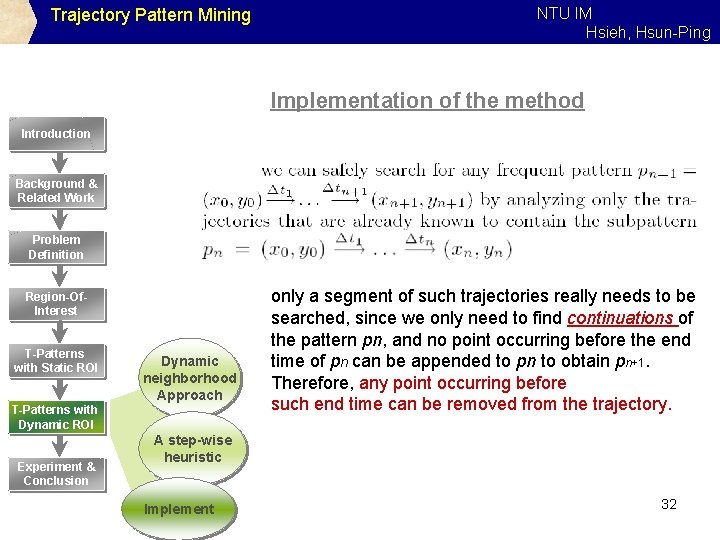Trajectory Pattern Mining NTU IM Hsieh, Hsun-Ping Implementation of the method Introduction Background & Related Work Problem Definition Region-Of. Interest T-Patterns with Static ROI Dynamic neighborhood Approach T-Patterns with Dynamic ROI Experiment & Conclusion only a segment of such trajectories really needs to be searched, since we only need to find continuations of the pattern pn, and no point occurring before the end time of pn can be appended to pn to obtain pn+1. Therefore, any point occurring before such end time can be removed from the trajectory. A step-wise heuristic Implement 32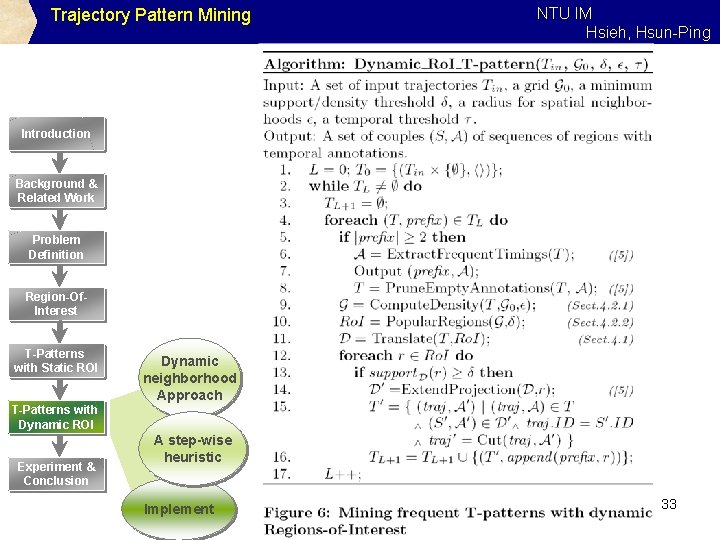Trajectory Pattern Mining NTU IM Hsieh, Hsun-Ping Introduction Background & Related Work Problem Definition Region-Of. Interest T-Patterns with Static ROI Dynamic neighborhood Approach T-Patterns with Dynamic ROI Experiment & Conclusion A step-wise heuristic Implement 33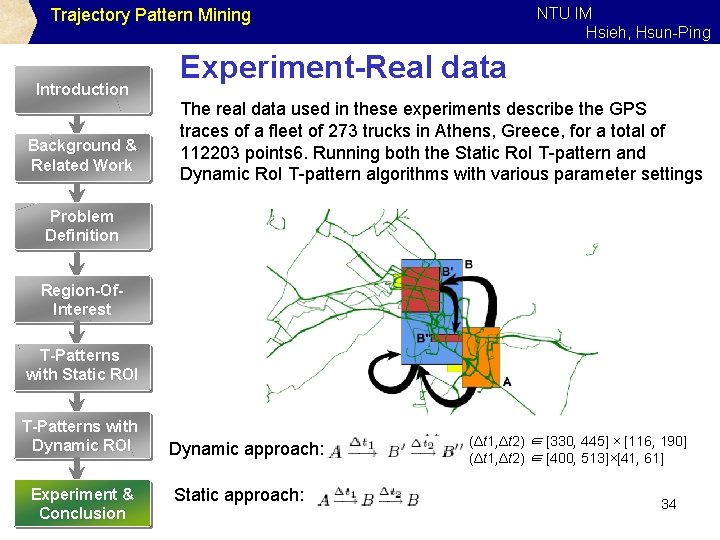NTU IM Hsieh, Hsun-Ping Trajectory Pattern Mining Introduction Background & Related Work Experiment-Real data The real data used in these experiments describe the GPS traces of a fleet of 273 trucks in Athens, Greece, for a total of 112203 points 6. Running both the Static Ro. I T-pattern and Dynamic Ro. I T-pattern algorithms with various parameter settings Problem Definition Region-Of. Interest T-Patterns with Static ROI T-Patterns with Dynamic ROI Experiment & Conclusion Dynamic approach: Static approach: (Δt 1, Δt 2) ∈ [330, 445] × [116, 190] (Δt 1, Δt 2) ∈ [400, 513]×[41, 61] 34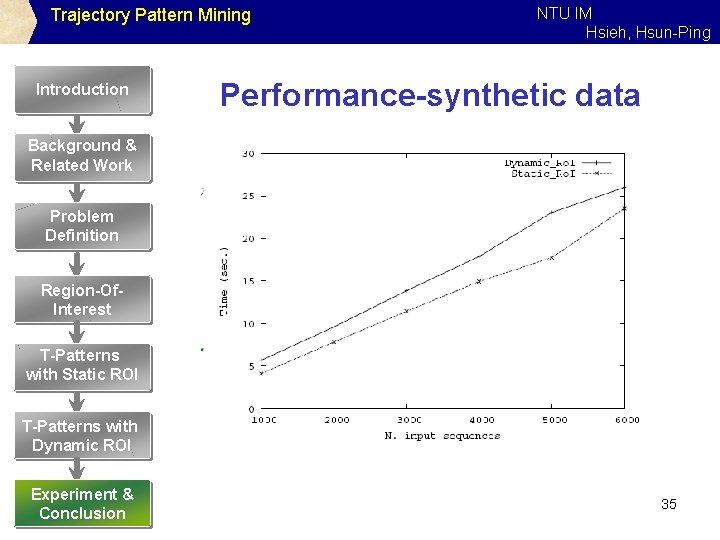Trajectory Pattern Mining Introduction NTU IM Hsieh, Hsun-Ping Performance-synthetic data Background & Related Work Problem Definition Region-Of. Interest T-Patterns with Static ROI T-Patterns with Dynamic ROI Experiment & Conclusion 35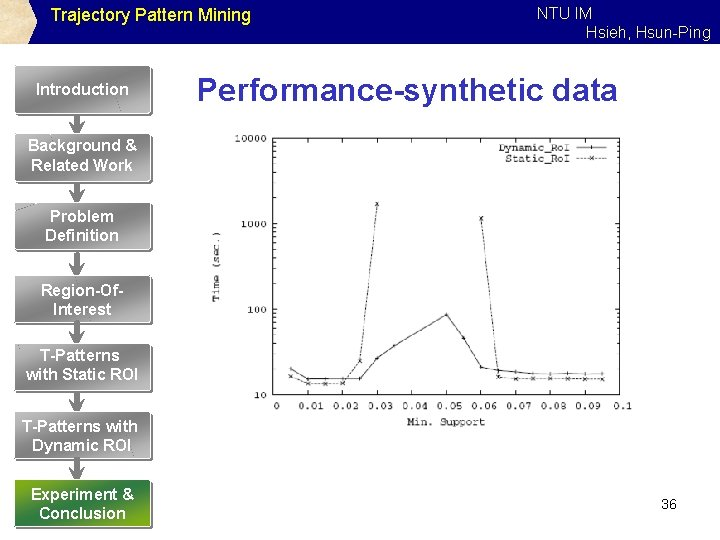Trajectory Pattern Mining Introduction NTU IM Hsieh, Hsun-Ping Performance-synthetic data Background & Related Work Problem Definition Region-Of. Interest T-Patterns with Static ROI T-Patterns with Dynamic ROI Experiment & Conclusion 36NTU IM Hsieh, Hsun-Ping Trajectory Pattern Mining Introduction Background & Related Work Problem Definition Region-Of. Interest Conclusion T-patterns are a basic building block for spatio-temporal data mining, around which more sophisticated analysis tools can be constructed, including: • integration with background geographic knowledge • adequate visualization metaphors for T-patterns • adequate mechanisms for spatio-temporal querying T-Patterns with Static ROI T-Patterns with Dynamic ROI Experiment & Conclusion 37